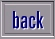Home Page Biology Chemistry Physics Science Superstars Inventions Teachers Stress Homework Help Zephyrus Training About Us Contact Us Credits Disclaimer Privacy Cookies

 Question:  Using the numbers 10, 1, 5, 4, 25 and 2, I need to make 983. You can use the numbers only once each but you can use these: add, subtract, divide and multiply as many times as you like. Answer:  Here is a suggested answer: Multiply 25 by 4 to get 100 Subtract 1 from 100 to get 99 Multiply 99 by 10 to get 990 Subtract 5 to get 985 Subtract 2 to get 983 (Your answer) (25 x 4) = (100 - 1) = (99 x 10) = (990 - 5) = (985 - 2) = 983 It's worth practising a lot of these on your own just to get the feel of the numbers. Brackets are very useful.to homework page